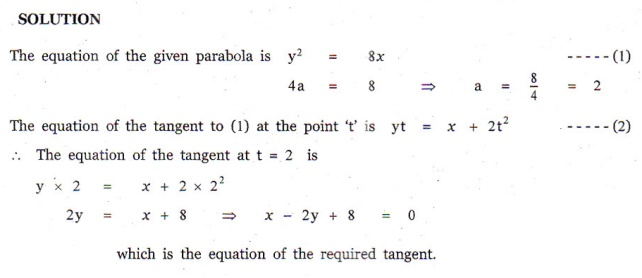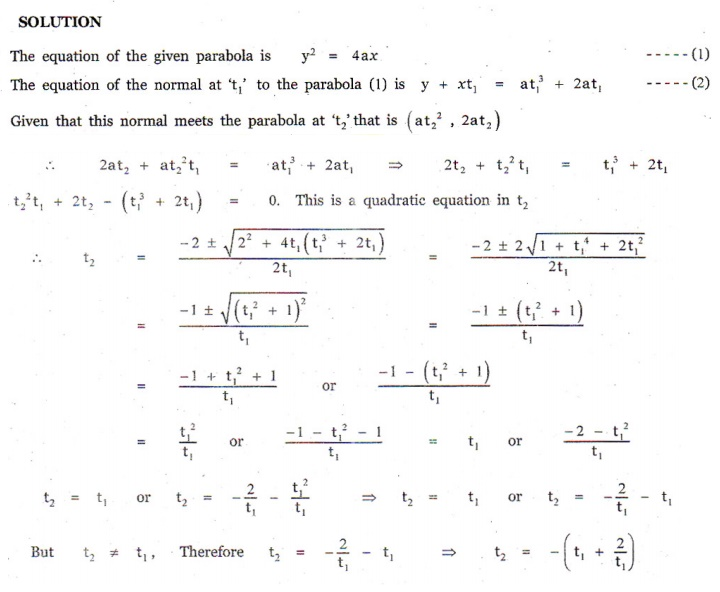Home | | Maths 12th Std | Exercise 5.4: Tangents and Normals to Conics

# Exercise 5.4: Tangents and Normals to Conics

Maths Book back answers and solution for Exercise questions - Find the equations of Tangents and Normals to Conics

EXERCISE 5.4

1. Find  the  equations  of  the  two  tangents that can be drawn from (5, 2) to the ellipse 2x+ 7 y= 14 .2. Find the equations of tangents to the hyperbolawhich are parallel to 10 3+ 9 = 0.3. Show that the line  + 4 = 0 is a tangent to the ellipse x+ 3y= 12 . Also find the coordinates of the point of contact.4. Find the equation of the tangent to the parabola y= 16perpendicular to 2+ 2 + 3 = 0 .5. Find the equation of the tangent at = 2 to the parabola y= 8. (Hint: use parametric form)6. Find the equations of the tangent and normal to hyperbola 12x 9 y= 108 at θ = π/3. (Hint: use parametric form)7. Prove that the point of intersection of the tangents at ‘ t’ and ‘ t’on the parabola y2 = 4ax is [at1t2 , a (t+ t2 )].8. If the normal at the point ‘ ’ on the parabola y= 4ax meets the parabola again at the point  ‘ t ’, then prove that t2= - ( t + 2/t1).